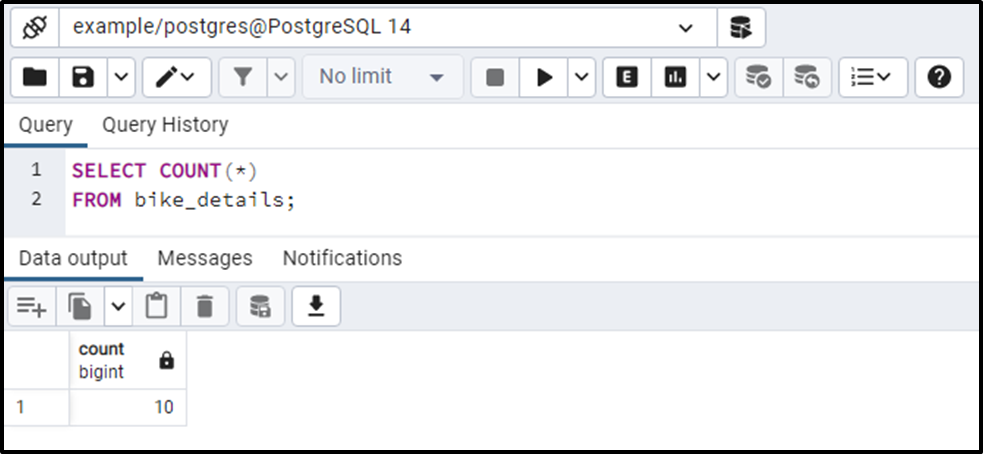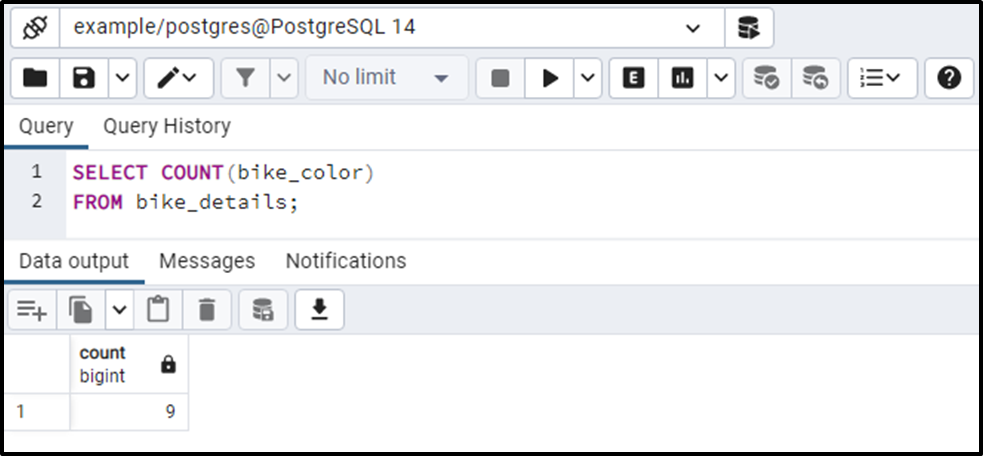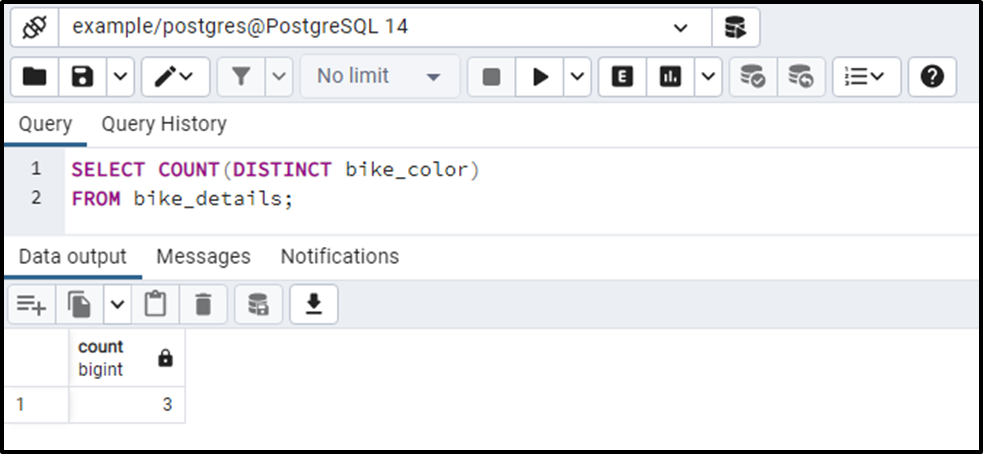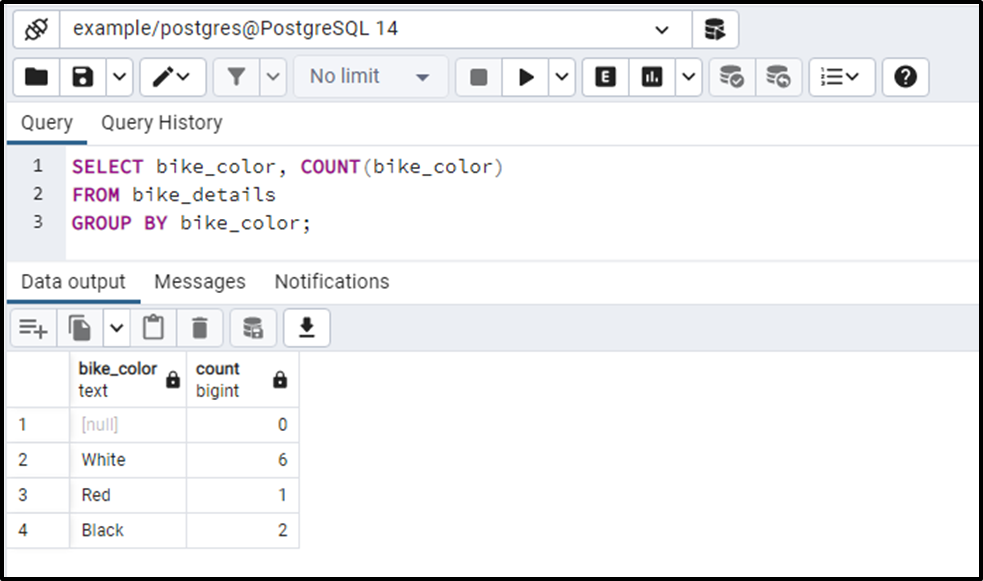##### How to Use COUNT() Function in PostgreSQL

The table rows can be counted using PostgreSQL's COUNT() function. It is an aggregate function that enables us to count the rows that meet the specified condition. There are multiple ways to use the COUNT() function, such as COUNT(*), COUNT(col_name), and COUNT(DISTINCT col_name). Each implementation of the COUNT() function serves a different functionality.

Let’s learn the working of the COUNT() function through Practical examples.

How to Use COUNT(*) Function in PostgreSQL?

In PostgreSQL, the COUNT(*) function is used to get all the rows, including duplicates and NULL. The below snippet illustrates the syntax of the Postgres COUNT(*) function:

```SELECT COUNT(*)
FROM tab_name;```

COUNT(*) function will fetch all the rows(including duplicates and NULL) from the targeted table based on the specified condition.

Example: How Does the COUNT(*) Function Work in PostgreSQL?

We have created a bike_details table that contains some redundant/duplicated data. Here are the details of the bike_details table:The bike_details table has some duplicates and null values. The following query will calculate the total number of rows in the bike_details table:

```SELECT COUNT(*)
FROM bike_details;```The output shows that the bike_details table has 10 rows. It proves that the COUNT(*) function counted all the rows, including the duplicates and null.

How to Use COUNT(col_name) Function in PostgreSQL?

In PostgreSQL, the COUNT(col_name) function skips the null values and returns the rest of the records, including duplicates. The below snippet depicts the syntax of the Postgres COUNT(col_name) function:

```SELECT COUNT(col_name)
FROM tab_name;```

The below-given example will provide you more clarity about the COUNT(col_name) function.

Example: How Does the COUNT(col_name) Function Work in PostgreSQL?

Let’s implement the COUNT(col_name) function on the bike_color column:

```SELECT COUNT(bike_color)
FROM bike_details;```The COUNT(col_name) function returns ‘9’ instead of ‘10’. It proves that the COUNT(col_name) function counted all the rows excluding the ‘null’.

How to Use COUNT(DISTINCT col_name) Function in Postgres?

In PostgreSQL, executing the COUNT() function along with the DISTINCT keyword will skip the duplicated records and return the unique ones only. The below snippet illustrates the syntax of the Postgres COUNT(DISTINCT col_name) function:

```SELECT COUNT(DISTINCT col_name)
FROM tab_name;```

Let’s comprehend the working of the COUNT(DISTINCT) function with the below-given example.

Example: How Does the COUNT(DISTINCT) Function Work in PostgreSQL?

Let’s execute the SELECT statement with COUNT(DISTINCT) function to count the unique records of the bike_color column:

```SELECT COUNT(DISTINCT bike_color)
FROM bike_details;```The output shows that there are only three distinct colors in the bike_color column.

How to Use the Postgres COUNT() Function With GROUP BY Clause?

Use the GROUP BY clause along with the COUNT() function to calculate the number of items for every group. For instance, grouping the bike colors and calculating the number of items for each group.

Example: How Does the COUNT() Function Work With the GROUP BY Clause in Postgres?

By using the GROUP BY clause, let's group the items in the bike_color column and calculate the number of items in each group using the COUNT() function:

```SELECT bike_color, COUNT(bike_color)
FROM bike_details
GROUP BY bike_color;```This is how you can calculate the number of items present in each group.

Similarly, you can use the Postgres WHERE clause or HAVING clause to count the rows based on a specific condition.

Conclusion

The table rows can be counted using PostgreSQL's COUNT() function. There are multiple ways to use the COUNT() function, such as COUNT(*), COUNT(col_name), and COUNT(DISTINCT col_name). The COUNT(*) function returns all the records, including duplicates and NULL, COUNT(col_name) returns all records excluding the NULL values, while the COUNT(DISTINCT col_name) returns only unique records. This write-up explained various implementations of the COUNT() function using different examples.